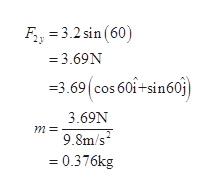# Using the same force table that you used, a student was given two forces and asked to find a single force that would produce equilibrium with the two forces. The two forces were given by providing one of the components and the angle the force made with the positive x- axis. The two forces were as follows: Force 1: ?? = −2.70 ?; ? = 220.0 degreees Force 2: ?? = 3.20 ?; ? = 60.0 degrees (a) For each force, calculate the magnitude of the force and the mass required to produce it. (b) Calculate the mass and its angle of application to produce equilibrium with these two forces. Please specify your angle as counterclockwise positive with respect to the positive x-axis.

Question
22 views

Using the same force table that you used, a student was given two forces and asked to find a single force that would produce equilibrium with the two forces. The two forces were given by providing one of the components and the angle the force made with the positive x- axis. The two forces were as follows: Force 1: ?? = −2.70 ?; ? = 220.0 degreees Force 2: ?? = 3.20 ?; ? = 60.0 degrees (a) For each force, calculate the magnitude of the force and the mass required to produce it. (b) Calculate the mass and its angle of application to produce equilibrium with these two forces. Please specify your angle as counterclockwise positive with respect to the positive x-axis.

check_circle

Step 1

a)

Force in x direction is f cosθ

Step 2

Force is given by the equation F=mg. Rearrange this equation and write in terms of m.

Step 3

Force in y direction i...help_outlineImage TranscriptioncloseF 3.2 sin (60) = 3.69N 3.69 cos 60i+sin60 3.69N m 9.8m/s = 0.376kg fullscreen

### Want to see the full answer?

See Solution

#### Want to see this answer and more?

Solutions are written by subject experts who are available 24/7. Questions are typically answered within 1 hour.*

See Solution
*Response times may vary by subject and question.
Tagged in

### Physics